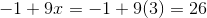# PSAT Math : How to find the nth term of an arithmetic sequence

## Example Questions

### Example Question #1 : How To Find The Nth Term Of An Arithmetic Sequence

2, 8, 14, 20

The first term in the sequence is 2, and each following term is determined by adding 6. What is the value of the 50th term?

300

302

296

320

296

Explanation:

We start by multiplying 6 times 46, since the first 4 terms are already listed. We then add the product, 276, to the last listed term, 20. This gives us our answer of 296.

### Example Question #2 : How To Find The Nth Term Of An Arithmetic Sequence

Which of the following could not be a term in the sequence 5, 10, 15, 20...?

3751

35

10005

2500

3751

Explanation:

All answers in the sequence must end in a 5 or a 0.

### Example Question #1 : How To Find The Nth Term Of An Arithmetic Sequence

In an arithmetic sequence, each term is two greater than the one that precedes it. If the sum of the first five terms of the sequence is equal to the difference between the first and fifth terms, what is the tenth term of the sequence?

–0.6

10

15.6

2.4

0.6

15.6

Explanation:

Let a1 represent the first term of the sequence and an represent the nth term.

We are told that each term is two greater than the term that precedes it. Thus, we can say that:

a2 = a1 + 2

a3 = a1 + 2 + 2 = a1 + 2(2)

a4 = a1 + 3(2)

a5 = a1 + 4(2)

an = a1 + (n-1)(2)

The problem tells us that the sum of the first five terms is equal to the difference between the fifth and first terms. Let's write an expression for the sum of the first five terms.

sum = a1 + (a1 + 2) + (a1 + 2(2)) + (a1 + 3(2)) + (a1 + 4(2))

= 5a1 + 2 + 4 + 6 + 8

= 5a1 + 20

Next, we want to write an expression for the difference between the fifth and first terms.

a5 - a1 = a1 + 4(2) – a1 = 8

Now, we set the two expressions equal and solve for a1.

5a1 + 20 = 8

Subtract 20 from both sides.

5a1 = –12

a1 = –2.4.

The question ultimately asks us for the tenth term of the sequence. Now, that we  have the first term, we can find the tenth term.

a10 = a1 + (10 – 1)(2)

a10 = –2.4 + 9(2)

= 15.6

### Example Question #1 : How To Find The Nth Term Of An Arithmetic Sequence

In a certain sequence, an+1 = (an)2 – 1, where an represents the nth term in the sequence. If the third term is equal to the square of the first term, and all of the terms are positive, then what is the value of (a2)(a3)(a4)?

48

72

24

63

6

48

Explanation:

Let a1 be the first term in the sequence. We can use the fact that an+1 = (an)2 – 1 in order to find expressions for the second and third terms of the sequence in terms of a1.

a2 = (a1)2 – 1

a3 = (a2)2 – 1 = ((a1)2 – 1)2 – 1

We can use the fact that, in general, (a – b)2 = a2 – 2abb2 in order to simplify the expression for a3.

a= ((a1)2 – 1)2 – 1

= (a1)4 – 2(a1)2 + 1 – 1 = (a1)4 – 2(a1)2

We are told that the third term is equal to the square of the first term.

a3 = (a1)2

We can substitute (a1)4 – 2(a1)for a3.

(a1)4 – 2(a1)= (a1)2

Subtract (a1)2 from both sides.

(a1)4 – 3(a1)= 0

Factor out (a1)from both terms.

(a1)2 ((a1)2 – 3) = 0

This means that either (a1)= 0, or (a1)2 – 3 = 0.

If (a1)= 0, then a1 must be 0. However, we are told that all the terms of the sequence are positive. Therefore, the first term can't be 0.

Next, let's solve (a1)2 – 3 = 0.

(a1)= 3

Take the square root of both sides.

a1 = ±√3

However, since all the terms are positive, the only possible value for a1 is √3.

Now, that we know that a1 = √3, we can find a2, a3, and a4.

a2 = (a1)2 – 1 = (√3)2 – 1 = 3 – 1 = 2

a3 = (a2)2 – 1 = 2– 1 = 4 – 1 = 3

a4 = (a3)2 – 1 = 32 – 1 = 9 – 1 = 8

The question ultimately asks for the product of the a2, a3, and a4, which would be equal to 2(3)(8), or 48.

### Example Question #1 : How To Find The Nth Term Of An Arithmetic Sequence

In the given sequence, the first term is 3 and each term after is one less than three times the previous term.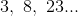What is the sixth term in the sequence?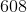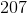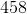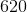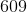Explanation:

The fourth term is: 3(23) – 1 = 69 – 1 = 68.

The fifth term is: 3(68) – 1 = 204 – 1 = 203.

The sixth term is: 3(203) – 1 = 609 – 1 = 608.

### Example Question #6 : How To Find The Nth Term Of An Arithmetic Sequence

Consider the following sequence of numbers: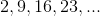What will be the 8th term in the sequence?

60

51

56

49

58

51

Explanation:

Each number in the sequence in 7 more than the number preceding it.

The equation for the terms in an arithmetic sequence is an = a1 + d(n-1), where d is the difference.

The formula for the terms in this sequence is therefore an = 2 + 7(n-1).

Plug in 8 for n to find the 8th term:

a8 = 2 + 7(8-1) = 51

### Example Question #1 : How To Find The Nth Term Of An Arithmetic Sequence

The second and fourth terms of an arithmetic sequence are 9 and 18, respectively. What is its first term?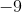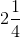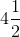Explanation:

The difference between the second and fourth terms of an arithmetic sequence is twice the common difference - or, equivalently, the common difference is half the difference between the second and fourth terms.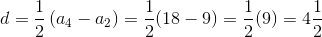The common difference can be subtracted from the second term to obtain the first term: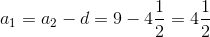### Example Question #2 : How To Find The Nth Term Of An Arithmetic Sequence

A sequence of numbers is represented by the equation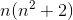, whererepresents theth term in the sequence. Which of the following equals the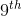term in the sequence?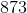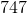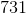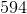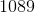Explanation:

Take the equation that represents theth term in the sequence and plug in the value of 9 for: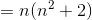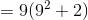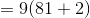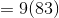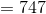The value of the 9th term is 747.

### Example Question #9 : How To Find The Nth Term Of An Arithmetic Sequence

You are given a sequence with the same difference between consecutive terms. We know it starts atand its 3rd term is. Find its 10th term.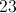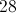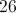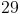From the given information, we know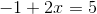, which means each consecutive difference is 3.